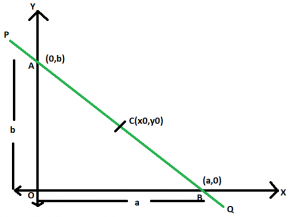# Equation of straight line passing through a given point which bisects it into two equal line segments

Given a straight line which passes through a given point (x0, y0) such that this point bisects the line segment in two equal line segments. The task is to find the equation of this straight line.

Examples:

Input: x0 = 4, y0 = 3
Output: 3x + 4y = 24

Input: x0 = 7, y0 = 12
Output: 12x + 7y = 168

## Recommended: Please try your approach on {IDE} first, before moving on to the solution.

Approach:Let PQ be the line and AB be the line segment between the axes. The x-intercept and y-intercept are a & b respectively.
Now, as C(x0, y0) bisects AB so,
x0 = (a + 0) / 2 i.e. a = 2x0
Similiarly, y0 = (0 + b) / 2 i.e. b = 2y0
We know that the equation of a straight line in intecept form is,

x / a + y / b = 1
Here, a = 2x0 & b = 2y0
So, x / 2x0 + y / 2y0 = 1
or, x / x0 + y / y0 = 2
Therefore, x * y0 + y * x0 = 2 * x0 * y0

Below is the implementation of the above approach:

## C++

 `// C++ implementation of the approach ` `#include ` `using` `namespace` `std; ` ` `  `// Function to print the equation ` `// of the required line ` `void` `line(``double` `x0, ``double` `y0) ` `{ ` `    ``double` `c = 2 * y0 * x0; ` `    ``cout << y0 << ``"x"` `         ``<< ``" + "` `<< x0 << ``"y = "` `<< c; ` `} ` ` `  `// Driver code ` `int` `main() ` `{ ` `    ``double` `x0 = 4, y0 = 3; ` `    ``line(x0, y0); ` ` `  `    ``return` `0; ` `} `

## Java

 `// Java implementation of the approach ` `class` `GFG ` `{ ` `     `  `// Function to print the equation ` `// of the required line ` `static` `void` `line(``double` `x0, ``double` `y0) ` `{ ` `    ``double` `c = (``int``)(``2` `* y0 * x0); ` `    ``System.out.println(y0 + ``"x"` `+ ``" + "` `+  ` `                       ``x0 + ``"y = "` `+ c); ` `} ` ` `  `// Driver code ` `public` `static` `void` `main(String[] args) ` `{ ` `    ``double` `x0 = ``4``, y0 = ``3``; ` `    ``line(x0, y0); ` `} ` `} ` ` `  `// This code is contributed  ` `// by Code_Mech `

## Python3

 `# Python 3 implementation of the approach ` ` `  `# Function to print the equation ` `# of the required line ` `def` `line(x0, y0): ` `    ``c ``=` `2` `*` `y0 ``*` `x0 ` `    ``print``(y0, ``"x"``, ``"+"``, x0, ``"y="``, c) ` ` `  `# Driver code ` `if` `__name__ ``=``=` `'__main__'``: ` `    ``x0 ``=` `4` `    ``y0 ``=` `3` `    ``line(x0, y0) ` `     `  `# This code is contributed by ` `# Surendra_Gangwar `

## C#

 `// C# implementation of the approach ` `using` `System; ` ` `  `class` `GFG ` `{ ` `     `  `// Function to print the equation ` `// of the required line ` `static` `void` `line(``double` `x0, ``double` `y0) ` `{ ` `    ``double` `c = (``int``)(2 * y0 * x0); ` `    ``Console.WriteLine(y0 + ``"x"` `+ ``" + "` `+  ` `                    ``x0 + ``"y = "` `+ c); ` `} ` ` `  `// Driver code ` `public` `static` `void` `Main(String[] args) ` `{ ` `    ``double` `x0 = 4, y0 = 3; ` `    ``line(x0, y0); ` `} ` `} ` ` `  `/* This code contributed by PrinciRaj1992 */`

## PHP

 ` `

Output:

```3x + 4y = 24
```

My Personal Notes arrow_drop_upCheck out this Author's contributed articles.

If you like GeeksforGeeks and would like to contribute, you can also write an article using contribute.geeksforgeeks.org or mail your article to contribute@geeksforgeeks.org. See your article appearing on the GeeksforGeeks main page and help other Geeks.

Please Improve this article if you find anything incorrect by clicking on the "Improve Article" button below.